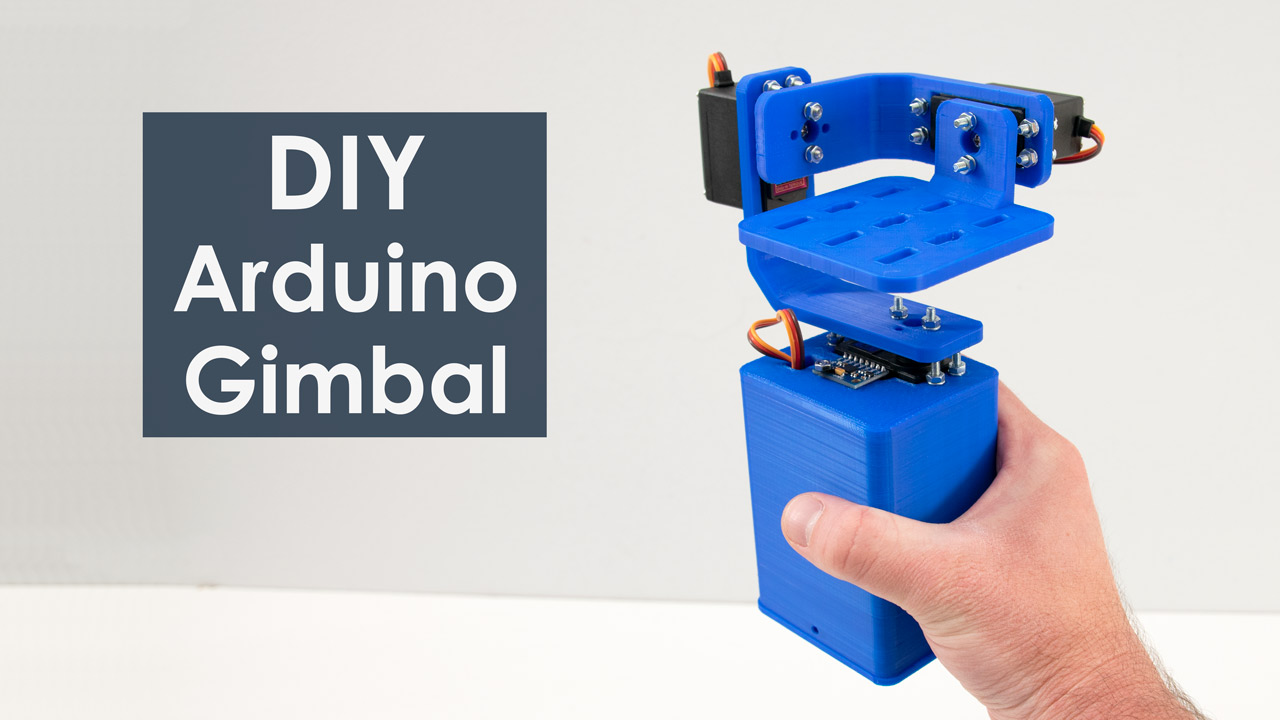# Arduino and MPU6050 Accelerometer and Gyroscope Tutorial

## Overview

In the first example, using the Processing development environment, we will make a 3D visualization of the sensor orientation, and in the second example we will make a simple self-stabilizing platform or a DIY Gimbal. Based on the MPU6050 orientation and its fused accelerometer and gyroscope data, we control the three servos that keep the platform level.

## How It Works

The MPU6050 IMU has both 3-Axis accelerometer and 3-Axis gyroscope integrated on a single chip.
The gyroscope measures rotational velocity or rate of change of the angular position over time, along the X, Y and Z axis. It uses MEMS technology and the Coriolis Effect for measuring, but for more details on it you can check my particular How MEMS Sensors Work tutorial. The outputs of the gyroscope are in degrees per second, so in order to get the angular position we just need to integrate the angular velocity.On the other hand, the MPU6050 accelerometer measures acceleration in the same way as explained in the previous video for the ADXL345 accelerometer sensor. Briefly, it can measure gravitational acceleration along the 3 axes and using some trigonometry math we can calculate the angle at which the sensor is positioned. So, if we fuse, or combine the accelerometer and gyroscope data we can get very accurate information about the sensor orientation.
The MPU6050 IMU is also called six-axis motion tracking device or 6 DoF (six Degrees of Freedom) device, because of its 6 outputs, or the 3 accelerometer outputs and the 3 gyroscope outputs.

## Arduino and MPU6050

Let’s take a look how we can connect and read the data from the MPU6050 sensor using the Arduino. We are using the I2C protocol for communication with the Arduino so we need only two wires for connecting it, plus the two wires for powering.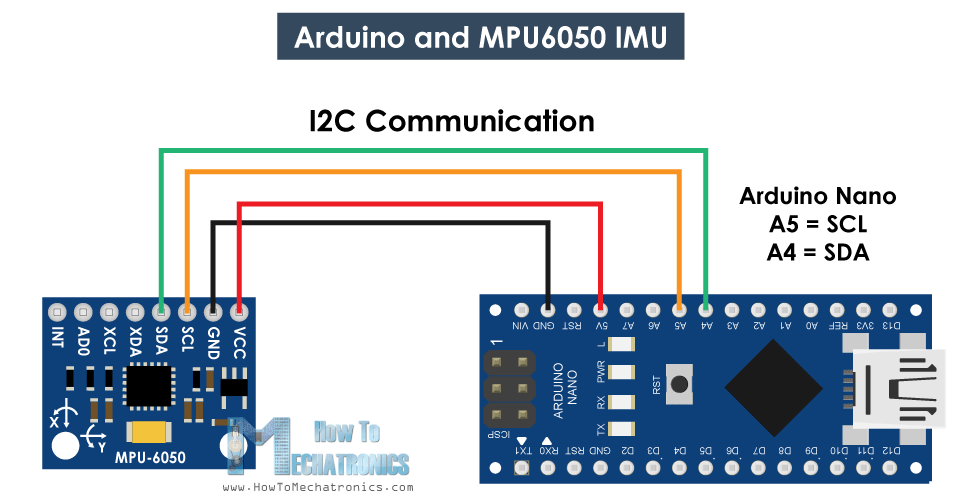You can get the components needed for this Arduino Tutorial from the links below:

## MPU6050 Arduino Code

Here’s the Arduino code for reading the data from the MPU6050 sensor. Below the code you can find a detail description of it.

1. /*
2. Arduino and MPU6050 Accelerometer and Gyroscope Sensor Tutorial
3. by Dejan, https://howtomechatronics.com
4. */
5. #include <Wire.h>
6. const int MPU = 0x68; // MPU6050 I2C address
7. float AccX, AccY, AccZ;
8. float GyroX, GyroY, GyroZ;
9. float accAngleX, accAngleY, gyroAngleX, gyroAngleY, gyroAngleZ;
10. float roll, pitch, yaw;
11. float AccErrorX, AccErrorY, GyroErrorX, GyroErrorY, GyroErrorZ;
12. float elapsedTime, currentTime, previousTime;
13. int c = 0;
14. void setup() {
15. Serial.begin(19200);
16. Wire.begin(); // Initialize comunication
17. Wire.beginTransmission(MPU); // Start communication with MPU6050 // MPU=0x68
18. Wire.write(0x6B); // Talk to the register 6B
19. Wire.write(0x00); // Make reset - place a 0 into the 6B register
20. Wire.endTransmission(true); //end the transmission
21. /*
22. // Configure Accelerometer Sensitivity - Full Scale Range (default +/- 2g)
23. Wire.beginTransmission(MPU);
24. Wire.write(0x1C); //Talk to the ACCEL_CONFIG register (1C hex)
25. Wire.write(0x10); //Set the register bits as 00010000 (+/- 8g full scale range)
26. Wire.endTransmission(true);
27. // Configure Gyro Sensitivity - Full Scale Range (default +/- 250deg/s)
28. Wire.beginTransmission(MPU);
29. Wire.write(0x1B); // Talk to the GYRO_CONFIG register (1B hex)
30. Wire.write(0x10); // Set the register bits as 00010000 (1000deg/s full scale)
31. Wire.endTransmission(true);
32. delay(20);
33. */
34. // Call this function if you need to get the IMU error values for your module
35. calculate_IMU_error();
36. delay(20);
37. }
38. void loop() {
39. // === Read acceleromter data === //
40. Wire.beginTransmission(MPU);
42. Wire.endTransmission(false);
43. Wire.requestFrom(MPU, 6, true); // Read 6 registers total, each axis value is stored in 2 registers
44. //For a range of +-2g, we need to divide the raw values by 16384, according to the datasheet
45. AccX = (Wire.read() << 8 | Wire.read()) / 16384.0; // X-axis value
46. AccY = (Wire.read() << 8 | Wire.read()) / 16384.0; // Y-axis value
47. AccZ = (Wire.read() << 8 | Wire.read()) / 16384.0; // Z-axis value
48. // Calculating Roll and Pitch from the accelerometer data
49. accAngleX = (atan(AccY / sqrt(pow(AccX, 2) + pow(AccZ, 2))) * 180 / PI) - 0.58; // AccErrorX ~(0.58) See the calculate_IMU_error()custom function for more details
50. accAngleY = (atan(-1 * AccX / sqrt(pow(AccY, 2) + pow(AccZ, 2))) * 180 / PI) + 1.58; // AccErrorY ~(-1.58)
51. // === Read gyroscope data === //
52. previousTime = currentTime; // Previous time is stored before the actual time read
53. currentTime = millis(); // Current time actual time read
54. elapsedTime = (currentTime - previousTime) / 1000; // Divide by 1000 to get seconds
55. Wire.beginTransmission(MPU);
56. Wire.write(0x43); // Gyro data first register address 0x43
57. Wire.endTransmission(false);
58. Wire.requestFrom(MPU, 6, true); // Read 4 registers total, each axis value is stored in 2 registers
59. GyroX = (Wire.read() << 8 | Wire.read()) / 131.0; // For a 250deg/s range we have to divide first the raw value by 131.0, according to the datasheet
62. // Correct the outputs with the calculated error values
63. GyroX = GyroX + 0.56; // GyroErrorX ~(-0.56)
64. GyroY = GyroY - 2; // GyroErrorY ~(2)
65. GyroZ = GyroZ + 0.79; // GyroErrorZ ~ (-0.8)
66. // Currently the raw values are in degrees per seconds, deg/s, so we need to multiply by sendonds (s) to get the angle in degrees
67. gyroAngleX = gyroAngleX + GyroX * elapsedTime; // deg/s * s = deg
68. gyroAngleY = gyroAngleY + GyroY * elapsedTime;
69. yaw = yaw + GyroZ * elapsedTime;
70. // Complementary filter - combine acceleromter and gyro angle values
71. roll = 0.96 * gyroAngleX + 0.04 * accAngleX;
72. pitch = 0.96 * gyroAngleY + 0.04 * accAngleY;
73. // Print the values on the serial monitor
74. Serial.print(roll);
75. Serial.print("/");
76. Serial.print(pitch);
77. Serial.print("/");
78. Serial.println(yaw);
79. }
80. void calculate_IMU_error() {
81. // We can call this funtion in the setup section to calculate the accelerometer and gyro data error. From here we will get the error values used in the above equations printed on the Serial Monitor.
82. // Note that we should place the IMU flat in order to get the proper values, so that we then can the correct values
83. // Read accelerometer values 200 times
84. while (c < 200) {
85. Wire.beginTransmission(MPU);
86. Wire.write(0x3B);
87. Wire.endTransmission(false);
88. Wire.requestFrom(MPU, 6, true);
93. AccErrorX = AccErrorX + ((atan((AccY) / sqrt(pow((AccX), 2) + pow((AccZ), 2))) * 180 / PI));
94. AccErrorY = AccErrorY + ((atan(-1 * (AccX) / sqrt(pow((AccY), 2) + pow((AccZ), 2))) * 180 / PI));
95. c++;
96. }
97. //Divide the sum by 200 to get the error value
98. AccErrorX = AccErrorX / 200;
99. AccErrorY = AccErrorY / 200;
100. c = 0;
101. // Read gyro values 200 times
102. while (c < 200) {
103. Wire.beginTransmission(MPU);
104. Wire.write(0x43);
105. Wire.endTransmission(false);
106. Wire.requestFrom(MPU, 6, true);
111. GyroErrorX = GyroErrorX + (GyroX / 131.0);
112. GyroErrorY = GyroErrorY + (GyroY / 131.0);
113. GyroErrorZ = GyroErrorZ + (GyroZ / 131.0);
114. c++;
115. }
116. //Divide the sum by 200 to get the error value
117. GyroErrorX = GyroErrorX / 200;
118. GyroErrorY = GyroErrorY / 200;
119. GyroErrorZ = GyroErrorZ / 200;
120. // Print the error values on the Serial Monitor
121. Serial.print("AccErrorX: ");
122. Serial.println(AccErrorX);
123. Serial.print("AccErrorY: ");
124. Serial.println(AccErrorY);
125. Serial.print("GyroErrorX: ");
126. Serial.println(GyroErrorX);
127. Serial.print("GyroErrorY: ");
128. Serial.println(GyroErrorY);
129. Serial.print("GyroErrorZ: ");
130. Serial.println(GyroErrorZ);
131. }

Code Description: So first we need to include the Wire.h library which is used for the I2C communication and define some variables needed storing the data.

In the setup section, we need initialize the wire library and reset the sensor through the power management register. In order to do that we need to take a look at the datasheet of the sensor from where we can see the register address.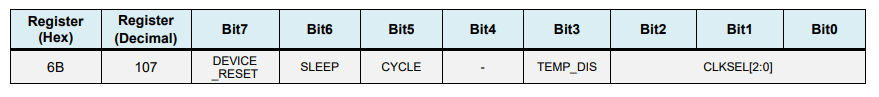Also, if we want, we can select the Full-Scale Range for the accelerometer and the gyroscope using their configuration registers. For this example, we will use the default +- 2g range for the accelerometer and 250 degrees/s range for the gyroscope, so I will leave this part of the code commented.

1. // Configure Accelerometer Sensitivity - Full Scale Range (default +/- 2g)
2. Wire.beginTransmission(MPU);
3. Wire.write(0x1C); //Talk to the ACCEL_CONFIG register (1C hex)
4. Wire.write(0x10); //Set the register bits as 00010000 (+/- 8g full scale range)
5. Wire.endTransmission(true);
6. // Configure Gyro Sensitivity - Full Scale Range (default +/- 250deg/s)
7. Wire.beginTransmission(MPU);
8. Wire.write(0x1B); // Talk to the GYRO_CONFIG register (1B hex)
9. Wire.write(0x10); // Set the register bits as 00010000 (1000deg/s full scale)
10. Wire.endTransmission(true);
11. */

In the loop section we start by reading the accelerometer data. The data for each axis is stored in two bytes or registers and we can see the addresses of these registers from the datasheet of the sensor.In order to read them all, we start with the first register, and using the requiestFrom() function we request to read all 6 registers for the X, Y and Z axes. Then we read the data from each register, and because the outputs are twos complement, we combine them appropriately to get the correct values.

1. // === Read acceleromter data === //
2. Wire.beginTransmission(MPU);
4. Wire.endTransmission(false);
5. Wire.requestFrom(MPU, 6, true); // Read 6 registers total, each axis value is stored in 2 registers
6. //For a range of +-2g, we need to divide the raw values by 16384, according to the datasheet
7. AccX = (Wire.read() << 8 | Wire.read()) / 16384.0; // X-axis value
8. AccY = (Wire.read() << 8 | Wire.read()) / 16384.0; // Y-axis value
9. AccZ = (Wire.read() << 8 | Wire.read()) / 16384.0; // Z-axis value

In order to get output values from -1g to +1g, suitable for calculating the angles, we divide the output with the previously selected sensitivity.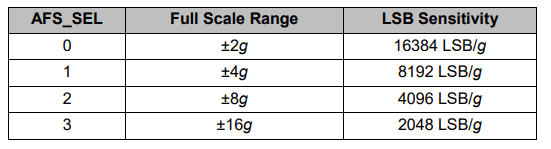Finally, using these two formulas, we calculate the roll and pitch angles from the accelerometer data.

1. // Calculating Roll and Pitch from the accelerometer data
2. accAngleX = (atan(AccY / sqrt(pow(AccX, 2) + pow(AccZ, 2))) * 180 / PI) - 0.58; // AccErrorX ~(0.58) See the calculate_IMU_error()custom function for more details
3. accAngleY = (atan(-1 * AccX / sqrt(pow(AccY, 2) + pow(AccZ, 2))) * 180 / PI) + 1.58; // AccErrorY ~(-1.58)

Next, using the same method we get the gyroscope data.We read the six gyroscope registers, combine their data appropriately and divide it by the previously selected sensitivity in order to get the output in degrees per second.

1. // === Read gyroscope data === //
2. previousTime = currentTime; // Previous time is stored before the actual time read
3. currentTime = millis(); // Current time actual time read
4. elapsedTime = (currentTime - previousTime) / 1000; // Divide by 1000 to get seconds
5. Wire.beginTransmission(MPU);
6. Wire.write(0x43); // Gyro data first register address 0x43
7. Wire.endTransmission(false);
8. Wire.requestFrom(MPU, 6, true); // Read 4 registers total, each axis value is stored in 2 registers
9. GyroX = (Wire.read() << 8 | Wire.read()) / 131.0; // For a 250deg/s range we have to divide first the raw value by 131.0, according to the datasheetHere you can notice that I correct the output values with some small calculated error values, which I will explain how we get them in a minute. So as the outputs are in degrees per second, now we need to multiply them with the time to get just degrees. The time value is captured before each reading iteration using the millis() function.

1. // Correct the outputs with the calculated error values
2. GyroX = GyroX + 0.56; // GyroErrorX ~(-0.56)
3. GyroY = GyroY - 2; // GyroErrorY ~(2)
4. GyroZ = GyroZ + 0.79; // GyroErrorZ ~ (-0.8)
5. // Currently the raw values are in degrees per seconds, deg/s, so we need to multiply by sendonds (s) to get the angle in degrees
6. gyroAngleX = gyroAngleX + GyroX * elapsedTime; // deg/s * s = deg
7. gyroAngleY = gyroAngleY + GyroY * elapsedTime;
8. yaw = yaw + GyroZ * elapsedTime;

Finally, we fuse the accelerometer and the gyroscope data using a complementary filter. Here, we take 96% of the gyroscope data because it is very accurate and doesn’t suffer from external forces. The down side of the gyroscope is that it drifts, or it introduces error in the output as the time goes on. Therefore, on the long term, we use the data from the accelerometer, 4% in this case, enough to eliminate the gyroscope drift error.

1. // Complementary filter - combine acceleromter and gyro angle values
2. roll = 0.96 * gyroAngleX + 0.04 * accAngleX;
3. pitch = 0.96 * gyroAngleY + 0.04 * accAngleY;

However, as we cannot calculate the Yaw from the accelerometer data, we cannot implement the complementary filter on it.
Before we take a look at the results, let me quickly explain how to get the error correction values.  For calculate these errors we can call the calculate_IMU_error() custom function while the sensor is in flat still position. Here we do 200 readings for all outputs, we sum them and divide them by 200. As we are holding the sensor in flat still position, the expected output values should be 0. So, with this calculation we can get the average error the sensor makes.

1. void calculate_IMU_error() {
2. // We can call this funtion in the setup section to calculate the accelerometer and gyro data error. From here we will get the error values used in the above equations printed on the Serial Monitor.
3. // Note that we should place the IMU flat in order to get the proper values, so that we then can the correct values
4. // Read accelerometer values 200 times
5. while (c < 200) {
6. Wire.beginTransmission(MPU);
7. Wire.write(0x3B);
8. Wire.endTransmission(false);
9. Wire.requestFrom(MPU, 6, true);
14. AccErrorX = AccErrorX + ((atan((AccY) / sqrt(pow((AccX), 2) + pow((AccZ), 2))) * 180 / PI));
15. AccErrorY = AccErrorY + ((atan(-1 * (AccX) / sqrt(pow((AccY), 2) + pow((AccZ), 2))) * 180 / PI));
16. c++;
17. }
18. //Divide the sum by 200 to get the error value
19. AccErrorX = AccErrorX / 200;
20. AccErrorY = AccErrorY / 200;
21. c = 0;
22. // Read gyro values 200 times
23. while (c < 200) {
24. Wire.beginTransmission(MPU);
25. Wire.write(0x43);
26. Wire.endTransmission(false);
27. Wire.requestFrom(MPU, 6, true);
32. GyroErrorX = GyroErrorX + (GyroX / 131.0);
33. GyroErrorY = GyroErrorY + (GyroY / 131.0);
34. GyroErrorZ = GyroErrorZ + (GyroZ / 131.0);
35. c++;
36. }
37. //Divide the sum by 200 to get the error value
38. GyroErrorX = GyroErrorX / 200;
39. GyroErrorY = GyroErrorY / 200;
40. GyroErrorZ = GyroErrorZ / 200;
41. // Print the error values on the Serial Monitor
42. Serial.print("AccErrorX: ");
43. Serial.println(AccErrorX);
44. Serial.print("AccErrorY: ");
45. Serial.println(AccErrorY);
46. Serial.print("GyroErrorX: ");
47. Serial.println(GyroErrorX);
48. Serial.print("GyroErrorY: ");
49. Serial.println(GyroErrorY);
50. Serial.print("GyroErrorZ: ");
51. Serial.println(GyroErrorZ);
52. }

We simply print the values on the serial monitor and once we know them, we can implement them in the code as shown earlier, for both the roll and pitch calculation, as well as for the 3 gyroscope outputs.Finally, using the Serial.print function we can print the Roll, Pitch and Yaw values on the serial monitor and see whether the sensor works properly.

## MPU6050 Orientation Tracking – 3D Visualization

Next, in order to make the 3D visualization example we just need accept this data the Arduino is sending through the serial port in the Processing development environment. Here’s the complete Processing code:

1. /*
2. Arduino and MPU6050 IMU - 3D Visualization Example
3. by Dejan, https://howtomechatronics.com
4. */
5. import processing.serial.*;
6. import java.awt.event.KeyEvent;
7. import java.io.IOException;
8. Serial myPort;
9. String data="";
10. float roll, pitch,yaw;
11. void setup() {
12. size (2560, 1440, P3D);
13. myPort = new Serial(this, "COM7", 19200); // starts the serial communication
14. myPort.bufferUntil('\n');
15. }
16. void draw() {
17. translate(width/2, height/2, 0);
18. background(233);
19. textSize(22);
20. text("Roll: " + int(roll) + " Pitch: " + int(pitch), -100, 265);
21. // Rotate the object
25. // 3D 0bject
26. textSize(30);
27. fill(0, 76, 153);
28. box (386, 40, 200); // Draw box
29. textSize(25);
30. fill(255, 255, 255);
31. text("www.HowToMechatronics.com", -183, 10, 101);
32. //delay(10);
33. //println("ypr:\t" + angleX + "\t" + angleY); // Print the values to check whether we are getting proper values
34. }
35. // Read data from the Serial Port
36. void serialEvent (Serial myPort) {
37. // reads the data from the Serial Port up to the character '.' and puts it into the String variable "data".
39. // if you got any bytes other than the linefeed:
40. if (data != null) {
41. data = trim(data);
42. // split the string at "/"
43. String items[] = split(data, '/');
44. if (items.length > 1) {
45. //--- Roll,Pitch in degrees
46. roll = float(items);
47. pitch = float(items);
48. yaw = float(items);
49. }
50. }
51. }

Here we read the incoming data from the Arduino and put it into the appropriate Roll, Pitch and Yaw variables. In the main draw loop, we use these values to rotate the 3D object, in this case that’s a simple box with a particular color and text on it.
If we run the sketch, we can see how good the MPU6050 sensor is for tracking orientation. The 3D object tracks the orientation of the sensor quite accurate and it’s also very responsive.As I mentioned, the only down side is that the Yaw will drift over time because we cannot use the complementary filter for it. For improving this we need to use an additional sensor. That’s usually a magnetometer which can be used as a long-term correction for the gyroscope Yaw drift. However, the MPU6050 actually have a feature that’s called Digital Motion Processor which is used for onboard calculations of the data and it’s capable of eliminating the Yaw drift.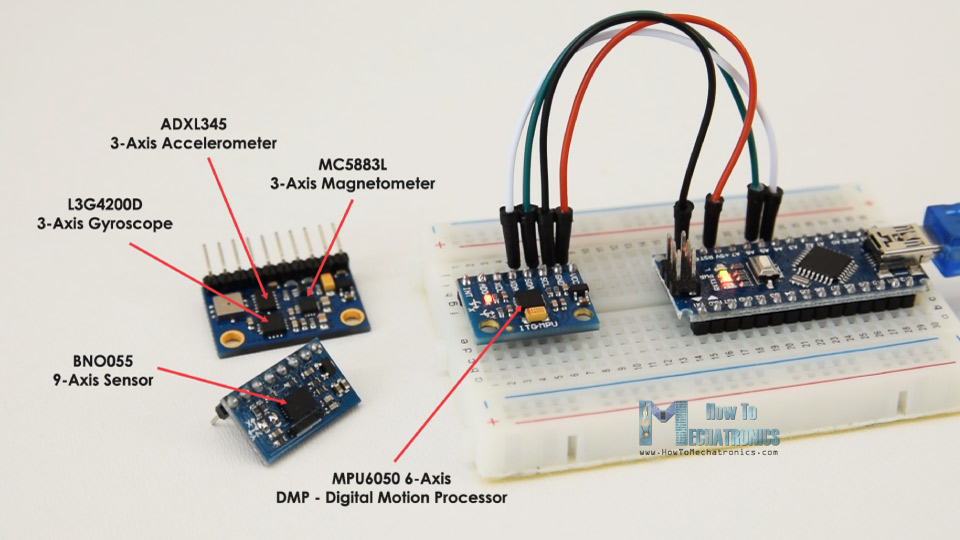Here’s the same 3D example with the Digital Motion Processor in use. We can see how accurate the orientation tracking is now, without the Yaw drift. The onboard processor can also calculate and output Quaternions which are used for representing orientations and rotations of objects in three dimensions. In this example we are actually using quaternions for representing the orientation which also doesn’t suffer from Gimbal lock which occurs when using Euler angles.Nevertheless, getting this data from the sensor is a bit more complicated than what we explained earlier. First of all, we need to connect and additional wire to an Arduino digital pin. That’s an interrupt pin which is used for reading this data type from the MPU6050.The code is also a bit more complicated so that’s why we are going to use the i2cdevlib library by Jeff Rowberg. This library can be downloaded from GitHub and I will include a link to in on the website article.
Once we install the library, we can open the MPU6050_DMP6 example from the library. This example is well explained with comments for each line.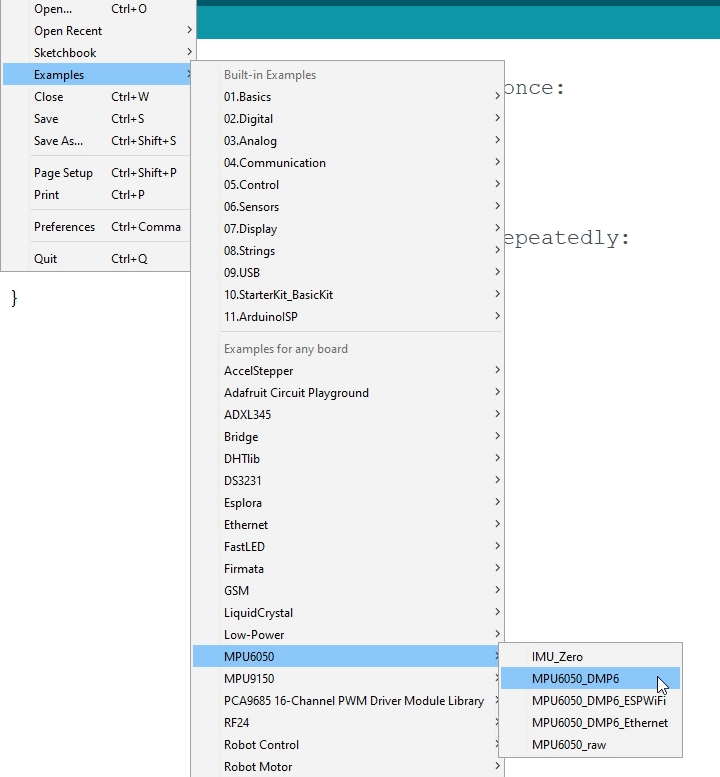Here we can select what kind of output we want, quaternions, Euler angles, yaw, pitch and roll, accelerations value or quaternions for the 3D visualization. This library also includes a Processing sketch for the 3D visualization example. I just modified it to get the box shape as in the previous example. Here’s the 3D visualization Processing code that works with the MPU6050_DPM6 example, for selected “OUTPUT_TEAPOT” output:

1. /*
2. Arduino and MPU6050 IMU - 3D Visualization Example
3. by Dejan, https://howtomechatronics.com
4. */
5. import processing.serial.*;
6. import java.awt.event.KeyEvent;
7. import java.io.IOException;
8. Serial myPort;
9. String data="";
10. float roll, pitch,yaw;
11. void setup() {
12. size (2560, 1440, P3D);
13. myPort = new Serial(this, "COM7", 19200); // starts the serial communication
14. myPort.bufferUntil('\n');
15. }
16. void draw() {
17. translate(width/2, height/2, 0);
18. background(233);
19. textSize(22);
20. text("Roll: " + int(roll) + " Pitch: " + int(pitch), -100, 265);
21. // Rotate the object
25. // 3D 0bject
26. textSize(30);
27. fill(0, 76, 153);
28. box (386, 40, 200); // Draw box
29. textSize(25);
30. fill(255, 255, 255);
31. text("www.HowToMechatronics.com", -183, 10, 101);
32. //delay(10);
33. //println("ypr:\t" + angleX + "\t" + angleY); // Print the values to check whether we are getting proper values
34. }
35. // Read data from the Serial Port
36. void serialEvent (Serial myPort) {
37. // reads the data from the Serial Port up to the character '.' and puts it into the String variable "data".
39. // if you got any bytes other than the linefeed:
40. if (data != null) {
41. data = trim(data);
42. // split the string at "/"
43. String items[] = split(data, '/');
44. if (items.length > 1) {
45. //--- Roll,Pitch in degrees
46. roll = float(items);
47. pitch = float(items);
48. yaw = float(items);
49. }
50. }
51. }

So here using the serialEvent() function we receive the quaternions coming from the Arduino, and in the main draw loop we use them to rotate the 3D object. If we run the sketch, we can see how good quaternions are for rotating objects in three dimensions.
In order not to overload this tutorial, I placed the second example, the DIY Arduino Gimbal or Self-Stabilizing platform,  on a separate article.